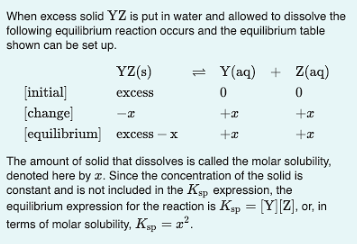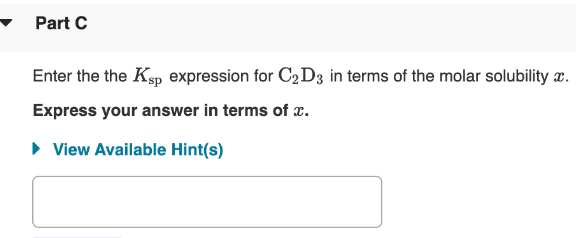# When excess solid YZ is put in water and allowed to dissolve the following equilibrium reaction occurs and the equilibrium table shown can be set up. The amount of solid that dissolves is called the molar solubility, denoted here by x. Since the concentration of the solid is constant and is not included in the Ksp expression, the equilibrium expression for the reaction is Ksp=[Y][Z], or, in terms of molar solubility, Ksp = x^2. Enter the Ksp expression for C2D3 in terms of the molar solubility x. Express your answer in terms of x .